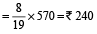### Partnership: Formulas, Tricks, Examples

Partnership, as the name suggests, its more than one person investing in something to increase resources, reduce risk etc.

The partners are liable for income/expenditure/profit/loss as per their percentage holding of the business or their partnership percentage. This is defined by their capital/work/other things contribution in terms of time. The partnership terms can be of many types, in questions you may encounter partners putting monies for different time periods, which can be calculated by multiplying the money with the time in months, the money has been invested for.

### Quicker Method to Solve the Questions

If the period of investment is the same for each partner, then the profit or loss is divided in the ratio of their investments.

If A and B are partners in a business, then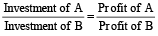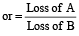If A, B and C are partners in a business, then

Investment of A : Investment of B : Investment of C

= Profit of A : Profit of B : Profit of C, or

= Loss of A : Loss of B : Loss of C

Example 1: A, B and C enter into a partnership by investing 1500, 2500 and 3000 rupees, respectively. A as manager gets one-tenth of the total profit and the remaining profit is divided among the three in the ratio of their investment. If A’s total share is Rs. 369, find the shares of B and C.

Solution: If total profit is x, then

A’s share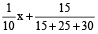of the balance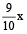⇒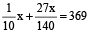⇒ 14x + 27x = 369 × 140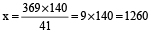B’s share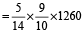= Rs. 405

C’s share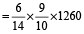= Rs. 486

If the period of investment is different, then the profit or loss is divided in the ratio of their Monthly Equivalent Investment.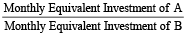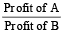or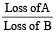i.e.,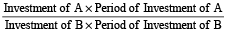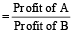orIf A, B and C are partners in a business, then:

Monthly Equivalent Investment of A : Monthly Equivalent Investment of B : Monthly Equivalent Investment of C

= Profit of A : Profit of B : Profit of C.

= Loss of A : Loss of B : Loss of C.

Example 2: Three persons A, B, C rent the grazing of a park for Rs. 570. A puts in 126 oxen in the park for 3 months, B puts in 162 oxen for 5 months and C puts in 216 oxen for 4 months. What part of the rent should each person pay?

Solution: Monthly equivalent rent of A = 126 × 3 = 378

Monthly equivalent rent of B = 162 × 5 = 810

Monthly equivalent rent of C = 216 × 4 = 864

∴ Rent is to be divided in the ratio 378 : 810 : 864,

ie. 7 : 15 : 16

∴  A would have to pay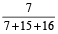of the rent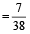of the rent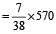= Rs. 105

∴ B would have to pay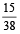of the rent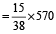= Rs. 225

and C would have to pay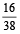,

i.e.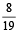of the rent